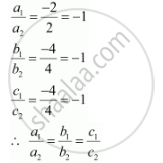Share

# Show that the Line Through the Points (4, 7, 8) (2, 3, 4) is Parallel to the Line Through the Points (−1, −2, 1), (1, 2, 5). - CBSE (Commerce) Class 12 - Mathematics

#### Question

Show that the line through the points (4, 7, 8) (2, 3, 4) is parallel to the line through the points (−1, −2, 1), (1, 2, 5).

#### Solution

Let AB be the line through the points, (4, 7, 8) and (2, 3, 4), and CD be the line through the points, (−1, −2, 1) and (1, 2, 5).

The directions ratios, a1b1c1, of AB are (2 − 4), (3 − 7), and (4 − 8) i.e., −2, −4, and −4.

The direction ratios, a2b2c2, of CD are (1 − (−1)), (2 − (−2)), and (5 − 1) i.e., 2, 4, and 4.

AB will be parallel to CD, if  a_1/a_2 = b_1/b_2=c_1/c_2Thus, AB is parallel to CD.

Is there an error in this question or solution?

#### APPEARS IN

NCERT Solution for Mathematics Textbook for Class 12 (2018 to Current)
Chapter 11: Three Dimensional Geometry
Q: 3 | Page no. 477

#### Video TutorialsVIEW ALL 

Solution Show that the Line Through the Points (4, 7, 8) (2, 3, 4) is Parallel to the Line Through the Points (−1, −2, 1), (1, 2, 5). Concept: Equation of a Line in Space.
S